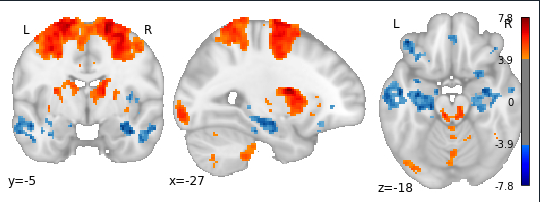# Colorbar in nilearn plot_stat_map cuts into brain when adding overlay

This is more of an aesthetic issue rather than a bug. I’m attempting to plot two contrasts in one image, using the following code:

``````cut_coordinates = [ -27, -5, -18]

thresh_h_img = nilearn.image.load_img("thresh_h_zstat1.nii.gz") # A > B
thresh_l_img = nilearn.image.load_img("thresh_l_zstat1.nii.gz") # B > A

display = nilearn.plotting.plot_stat_map(thresh_h_img,
display_mode="ortho",
bg_img=bg_img,
black_bg=False,
draw_cross=False,
annotate=True,
colorbar=True,
cmap="jet",
cut_coords=cut_coordinates,
threshold=4.05,
symmetric_cbar=True,
)

``````

This results in the following image:I’ve been having trouble preventing the colorbar from encroaching into the z-slice, and was wondering whether there’s a way to prevent this in nilearn or if this is handled in `matplotlib`?

Hi @dlevitas,

This is just a matplotlib thing. You can create your own bigger figure/axes instance using matplotlib and then pass it in to the `plot_stat_map` function with the `figure` / `axes` argument.

Best,
Steven

Hi @Steven,

Can you clarify how you do that? I’ve tried inserting a blank figure with different sizes, but the colorbar issues continues once I set the `display.add_overlay()`.

``````cut_coordinates = [ -27, -5, -18]

thresh_h_img = nilearn.image.load_img("thresh_h_zstat1.nii.gz") # A > B
thresh_l_img = nilearn.image.load_img("thresh_l_zstat1.nii.gz") # B > A

display = nilearn.plotting.plot_stat_map(thresh_h_img,
display_mode="ortho",
bg_img=bg_img,
black_bg=False,
draw_cross=False,
annotate=True,
colorbar=True,
cmap="jet",
cut_coords=cut_coordinates,
threshold=4.05,
symmetric_cbar=True,
figure=fig
)